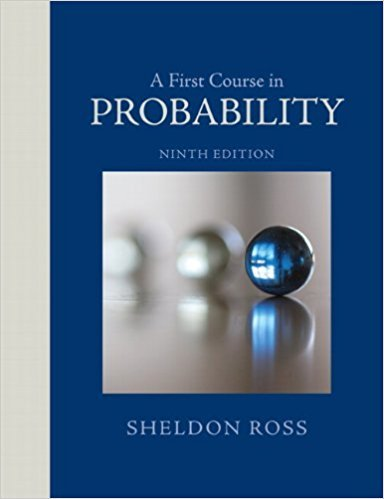×
Get Full Access to A First Course In Probability - 9 Edition - Chapter 2 - Problem 36p
Get Full Access to A First Course In Probability - 9 Edition - Chapter 2 - Problem 36p

×

# Two cards are chosen at random from a deck of 52 playingISBN: 9780321794772 63

## Solution for problem 36P Chapter 2

A First Course in Probability | 9th Edition

• Textbook Solutions
• 2901 Step-by-step solutions solved by professors and subject experts
• Get 24/7 help from StudySoup virtual teaching assistantsA First Course in Probability | 9th Edition

4 5 1 431 Reviews
10
3
Problem 36P

Problem 36P

Two cards are chosen at random from a deck of 52 playing cards. What is the probability that they

(a) are both aces?

(b) have the same value?

Step-by-Step Solution:

Solution:

Step 1 of 3:

It is given that a deck of cards has 52 cards and two cards have to be selected at random from the deck of cards.

Using this we need to find the required values.

Step 2 of 3

Step 3 of 3

##### ISBN: 9780321794772

Since the solution to 36P from 2 chapter was answered, more than 276 students have viewed the full step-by-step answer. A First Course in Probability was written by and is associated to the ISBN: 9780321794772. The answer to “Two cards are chosen at random from a deck of 52 playing cards. What is the probability that they(a) are both aces?________________(b) have the same value?” is broken down into a number of easy to follow steps, and 26 words. The full step-by-step solution to problem: 36P from chapter: 2 was answered by , our top Statistics solution expert on 08/11/17, 08:57AM. This textbook survival guide was created for the textbook: A First Course in Probability , edition: 9. This full solution covers the following key subjects: Cards, Deck, aces, chosen, both. This expansive textbook survival guide covers 4 chapters, and 469 solutions.

Unlock Textbook Solution